# Height to the base

The triangle area is 35 cm ^ 2. The size of the base is 10 cm. Find the length of height to the base.

Correct result:

v =  7 cm

#### Solution: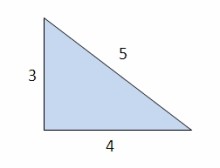We would be pleased if you find an error in the word problem, spelling mistakes, or inaccuracies and send it to us. Thank you!Tips to related online calculators

#### You need to know the following knowledge to solve this word math problem:

We encourage you to watch this tutorial video on this math problem:

## Next similar math problems:

• The dough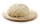The dough contains water, flour, and sugar. Water and flour in a ratio of 2: 3, flour, and sugar in a ratio of 2: 1. Determine the ratio of all three components of the dough.
• Thousand ballsWe have to create a thousand balls from a sphere with a diameter of 1 m. What will be their radius?
• Average priceSixth grade went on a trip to Moravia. Each of the 26 pupils paid CZK 320 and the school paid a total of CZK 3,510. What was the average price of a trip per student?
• Water tank300hl of water was filled into the tank 12 m long and 6 m wide. How high does it reach?
• Telco company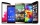The upstairs communications company offers customers a special long distance calling rate that includes a \$0.10 per minute charge. Which of the following represents this fee scheduale where m represents the number of minutes and c is the overall cost of t
• LibraryNew books were purchased for the library. Five-eighths were professional books, one-fifth were encyclopedias, and 231 books were dictionaries. How many professional books were there?
• Running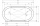The length of the inner edge of the running oval is 400 m. The straight sections measure 90 m. Calculate the dimensions of the oval - the rectangle where this oval can fit.
• Edge cFind the edge c of cuboid if an edge a = 20 mm, b = 30 mm and surface area S = 8000 mm2.
• Cylinder containerThe cylindrical container with a diameter of 1.8 m contains 2,000 liters of water. How high does the water reach?
• Aquarium heightHow high does the water in the aquarium reach, if there are 36 liters of water in it? The length of the aquarium is 60 cm and the width is 4 dm.
• What is bigger?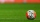Which ball has a larger volume: a football with a circumference of 66 cm or a volleyball with a diameter of 20 cm?
• Volume per timeHow long does fill take for a pump with a volume flow of 200 l per minute fill a cube-shaped tank up to 75% of its height if the length of the cube edge is 4 m?
• Second sideCalculate the length of the other side of the rectangle if its circumference is 60 cm and one side is 10 cm long.
• The angles ratioThe angles in the ABC triangle are in the ratio 1: 2: 3. find the sizes of the angles and determine what kind of a triangle it is.
• Cylindrical tank9.6 hl of water is poured into a cylindrical tank with a bottom diameter of 1.2 m. What height in centimeters does the water reach?
• Height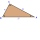The content of the triangle is 35 cm2. The length of the base is 10 cm. Determine the length of the height on the base.
• Four prismsQuestion No. 1: The prism has the dimensions a = 2.5 cm, b = 100 mm, c = 12 cm. What is its volume? a) 3000 cm2 b) 300 cm2 c) 3000 cm3 d) 300 cm3 Question No.2: The base of the prism is a rhombus with a side length of 30 cm and a height of 27 cm. The heig